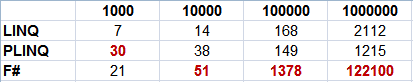# Performance Test – Prime numbers with LINQ vs PLINQ vs F#

Having spent quite a bit of time coding in F# recently I have thoroughly enjoyed the experience of coding in a functional style and come to really like the fact you can do so much with so little code.

One of the counter-claims against F# has always been the concerns over performance in the most performance critical applications, and with that in mind I decided to do a little experiment of my own using C# (LINQ & PLINQ) and F# to generate all the prime numbers under a given max value.

The LINQ and PLINQ methods in C# look something like this:

```private static void DoCalcSequentially(int max)
{
var numbers = Enumerable.Range(3, max-3);
var query =
numbers
.Where(n => Enumerable.Range(2, (int)Math.Sqrt(n))
.All(i => n % i != 0));
query.ToArray();
}

private static void DoCalcInParallel(int max)
{
var numbers = Enumerable.Range(3, max-3);
var parallelQuery =
numbers
.AsParallel()
.Where(n => Enumerable.Range(2, (int)Math.Sqrt(n))
.All(i => n % i != 0));
parallelQuery.ToArray();
}
```

The F# version on the other hand uses the fairly optimized algorithm I had been using in most of my project euler solutions:

```let mutable primeNumbers = 

// generate all prime numbers under <= this max
let getPrimes max =
// only check the prime numbers which are <= the square root of the number n
let hasDivisor n =
|> Seq.takeWhile (fun n' -> n' <= int(sqrt(double(n))))
|> Seq.exists (fun n' -> n % n' = 0)

// only check odd numbers <= max
let potentialPrimes = Seq.unfold (fun n -> if n > max then None else Some(n, n+2)) 3
// populate the prime numbers list
for n in potentialPrimes do if not(hasDivisor n) then primeNumbers <- primeNumbers @ [n]

```

Here’s the average execution time in milliseconds for each of these methods over 3 runs for max = 1000, 10000, 100000, 1000000:Have to admit this doesn’t make for a very comfortable reading…on average the F# version, despite being optimized, runs over 3 – 6 times as long as the standard LINQ version! The PLINQ version on the other hand, is slower in comparison to the standard LINQ version when the set of data is small as the overhead of partitioning, collating and coordinating the extra threads actually slows things down, but on a larger dataset the benefit of parallel processing starts to shine through.

#### UPDATE 13/11/2010:

Thanks for Jaen’s comment, the cause for the F# version of the code to be much slower is because of this line:

```primeNumbers <- primeNumbers @ [n]
```

because a new list is constructed every time and all elements from the previous list copied over.

Unfortunately, there’s no way to add an element to an existing List or Array in F# without getting a new list back (at least I don’t know of a way to do this), so to get around this performance handicap the easiest way is to make the prime numbers list a generic List instead (yup, luckily you are free to use CLR types in F#):

```open System.Collections.Generic

// initialize the prime numbers list with 2
let mutable primeNumbers = new List<int>()

// as before
...

// populate the prime numbers list

```

With this change, the performance of the F# code is now comparable to that of the standard LINQ version.

### 3 thoughts on “Performance Test – Prime numbers with LINQ vs PLINQ vs F#”

1.I would guess that this line:

2.3.